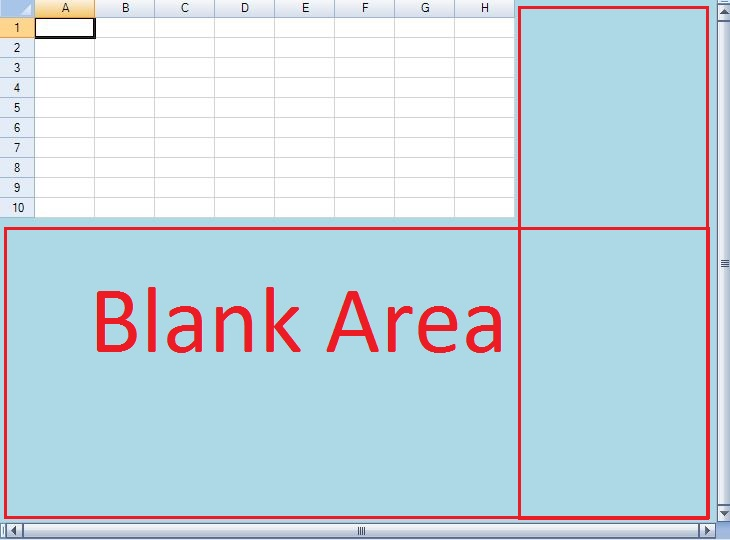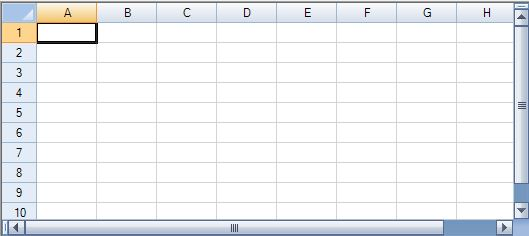﻿ How to: AutoSize Spread for WinForms# How to: AutoSize Spread for WinForms

The AutoSize feature of Spread COM removes the blank area around the grid cells such that Spread always takes the size of Rows and Column count. Unfortunately, this feature is not available as a built-in feature with Spread for WinForms. In this blog, we will discuss the an easy and simple implementation of AutoSize feature, ie, remove the blank area as shown in the image :# The Implementation

All we need to do is to calculate and sum the height and width of each element in SpreadSheet and set it to SpreadSheet's height and width. It involves following steps :

1. Calculate Height of all Rows, ColumnHeader and Horizontal Scrollbar
2. Calculate Width of all Columns, RowHeader and Vertical Scrollbar
3. Set Spreadsheet's Height and Width to the above calculated Height & Width

## Step 1 : Calculate Height

Height of all the Rows

``````
{
h = h +(int)r.Height;     //h is a global variable
}

``````

``````
{
h = h +(int) r.Height;
}

``````

Height of Horizontal Scrollbar

``````
hs = SystemInformation.HorizontalScrollBarHeight;

``````

It gives you the total height that Spread needs to show all these elements but no blank area.

``````
totalHeight=h+hs;

``````

## Step 2 : Calculate Width

Width of all Columns

``````
{
w =w +(int) c.Width; //w is the global variable
}

``````

``````
{
w = w + (int)c.Width;
}

``````

Width of Vertical ScrollBar

``````
vs = SystemInformation.VerticalScrollBarWidth;

``````

## Step 3 : Set the Size of SpreadSheet

Sum up the width for all elements to get the final width which Spread requires to show all these elements and hide the blank area.

``````
totalWidth=w+vs;

``````

Now all we need to do is to set the final height and width to Spread's height and width.

``````
Final output of this is similar to the one shown in image below:Above image shows that Spread does not contain any GrayArea for same number of Rows and Columns now. Download the sample application which demonstrate the above implementation more precisely. Download C# Sample Download VB.Net Sample